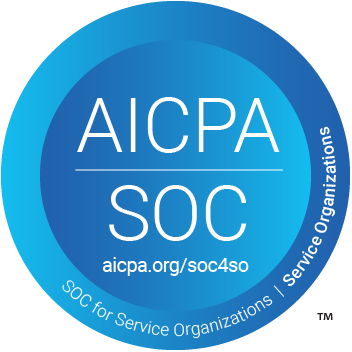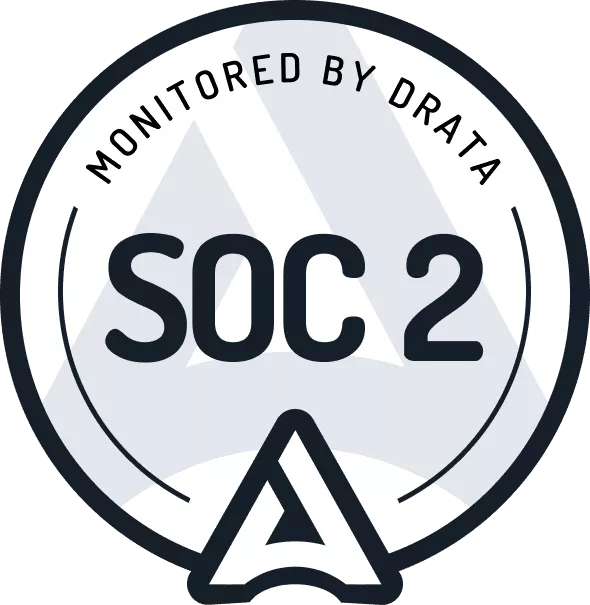# How to Calculate a Lease Liability and Lease Asset Under GASB 87 with Journal Entries

Lucas Russell | 2023-06-15

## GASB 87 Brings All Leases on Balance Sheet for Lessees

The Governmental Accounting Standards Board (GASB) has released a new lease accounting standard that replaces:

• GASB Statement No. 62: Issued in 2010, GASB 62 mirrors most of ASC 840 and harmonized the accounting between the lease accounting standards.
• GASB Statement No. 13: Issued in 1990 and outlined how to accounting for operating leases.
• NCGA Statement No. 5: Issued in 1986

The changes brought with GASB 87 are consistent with the other new lease accounting standards released by FASB – ASC 842 and IASB – IFRS 16.

The new lease accounting standard has been introduced to provide more information to users of financial statements. With a lease liability and lease asset recognized on balance it gives users far better insight into an organization's lease obligations.

## What Are the Changes

1. All leases come on balance sheet
2. No more lease classification, no more capital and operating leases, if the contract meets the definition of a lease for the lessee, it’s a lease. This is a major difference to ASC 842, where FASB decided to keep the lease classification

## How to Account for a Lease Under GASB 87

If you have experience accounting for a Capital lease under GASB 62 you will understand the required accounting is a lot more demanding than an operating lease. That’s because with a capital lease, the lessee must:

• Calculate the present value of the future lease payments and recognize a liability on the balance sheet
• Calculate the asset side of the lease, and recognize this amount on the balance sheet
• If the known future lease payments change, the lessee must reflect this in the lease

Now under GASB 87 all leases follow the accounting principles that are similar to a capital lease under GASB 82.

In this article, we will explore two practical examples of how to calculate leases as a lessee under GASB 87. The first example will focus on the initial recognition of lease liability and lease asset, which involves calculating the values of these components when there are no subsequent changes to the lease. In the second example, we will cover how to account for a lease modification, which occurs after the lease has commenced. We will highlight how modifying the lease can impact the value of the lease liability and lease asset.

It is important to note that this article assumes you have a good understanding of the principles of time value of money and present value techniques. If you are unsure about these concepts, we highly recommend reading our article "How to Calculate the Present Value of Future Lease Payments" as everything in GASB 87 is based on the idea of present valuing a set of future payments.

## Where to Start - The Lease Liability

A lease agreement can be very long and convoluted. From a lease accounting perspective, three main inputs need to be extracted to be able to calculate the net present value calculation of the lease liability:

1. The commencement of the payments, aka the commencement date of the lease
2. The payments required over the lease life, aka the lease term
3. The end date of the lease payments also referred to as the expiry of the lease

These three inputs determine the future lease payments, which the lessee is required to present value.

The final input is the discount rate. The discount rate is the input used to calculate the present value of the known future lease payments. This figure is not extracted from the lease agreement.

Calculating the net present value of a lease liability can be a complex process, as lease agreements can be lengthy and intricate. However, from a lease accounting perspective, there are three primary inputs that must be extracted to perform this calculation:

1. The commencement and expiry date of the lease, known as the lease term
2. The payment amounts during the lease term

These two inputs together determine the future lease payments, which the lessee must then present value.

In addition, to these two inputs, the final piece of information needed is the discount rate. This rate is used to calculate the present value of the known future lease payments. It is important to note that the discount rate is not typically included in the lease agreement itself and must be obtained separately.

Lease term

When accounting for a lease under GASB 87, there are several important factors to consider. Two critical aspects that require careful consideration are the commencement date and lease end date. Here's a breakdown of what you need to know:

• Commencement date: The commencement date of a lease is the start date of the lease term. This date is important because it's used to determine when the lease liability and lease asset should be recognized. The commencement date can be a judgment call and may differ from the lease signing date.
• Lease end date: Similar to the commencement date, determining the end date of a lease requires judgment. The lessee is responsible for selecting the most reasonably likely end date of the lease. This may or may not include renewals. It's important to consider all options available under the lease agreement to determine the lease end date accurately.

For more detailed guidance on accounting for a lease under GASB 87, you may refer to GASB 87.12.

Lease payments

With lease payments, there are three types of lease payments:

Lease Payment Type Description Include in Lease Liability Calculation
Fixed payments These payments are known at the inception of the lease. They are the payments made by a lessee to a lessor for the right to use an underlying asset during the lease term. This will typically consist of a regular payment based on a specific frequency such as monthly, quarterly, or annually. If a company were reasonably likely to exercise a purchase option within the contract which incurred a payment. You would include that payment as well. Yes
Variable Lease Payments Variable lease payments: these are payments that depend on an index or a rate, initially measured using the index or rate as at the commencement date. Common examples include the increase in fixed payments with the consumer price index (CPI) or market rent review (MRR) at a stipulated point in time. Yes
Truly Variable Lease Payments Variable lease payments that depend on sales or usage of the underlying asset are excluded from the lease liability. Instead, these payments are recognized in profit or loss in the period in which they occur. No

For further details, refer to GASB 87.21

Discount rate

When calculating the present value of lease payments, the interest rate implicit in the lease should be used if it can be determined with ease. However, if the implicit rate cannot be readily determined, the lessee must use their own incremental borrowing rate. It is important to exercise judgment in choosing the discount rate and be prepared to justify the rate used. For additional information on this topic, please refer to the following resource.

## Lease Asset

The value of the lease asset is primarily determined by the lease liability value. However, when first recognizing the value of the lease asset, there are other inputs that can impact its value. These inputs include:

• Direct costs incurred during the initiation of the lease.
• Lease payments made at or before the lease commencement date, less any lease incentives received.

These additional inputs must be taken into account when determining the overall value of the lease asset.For further details, refer to GASB 87 paragraph 31.

Once you have determined these values, the time has come to calculate the lease liability and lease asset in accordance with GASB 87.

## Modification accounting

It's crucial to emphasize the concept of modification accounting before delving deeper into this topic. Modification accounting refers to a situation where one or more inputs that are used to calculate the lease liability undergo a change. These inputs will either be lease payments or the lease term or both. Such changes can occur due to two reasons:

a) A modification to the terms and conditions of the contract

b) The lessee's decision to exercise an option in the contract, such as a renewal option.

Whenever such changes occur, the lessee must recalculate both the lease liability and the right of use asset. However, modification accounting can become complicated, especially when dealing with a decrease in the scope of the calculation.

In Example 2, we will demonstrate how to account for the lease liability and lease asset in a lease modification scenario.

## Practical examples

These examples will take you through from scratch how to calculate the lease liability and the right of use asset. To be able to follow the examples, there are some prerequisites.

• You understand the time value of money and how to calculate the present value. If you’re unsure of this, refer here.
• You understand when entering a lease, the lessee recognizes a lease liability and right of use asset.
• The lease liability will then unwind to zero upon completion of the lease, as there are no more lease payments.
• The right of use asset will be depreciated to zero based on the useful life of the leased asset. This may or may not be the same date as the accounting lease end date.

The calculation methodology we will use to calculate the lease liability is the XNPV function within excel. This present value function requires to inputs:

• The date of the payment
• The amount of the payment

Because lease payments can occur on an ad hoc basis, calculations will be performed daily. This is to ensure ultimate flexibility, especially if a lease modification occurs. In addition, the upfront time investment is comparable to performing a monthly calculation which will not nearly be as accurate or as flexible as a daily calculation.

If you would like a copy of the calculation, reach out to [email protected]. Following along with the calculation will make it a lot easier to grasp the concepts and use them as a template for your lease calculations.

So now you’re ready to calculate the lease liability and lease asset.

# Example 1 - Calculate the Lease Liability and Lease Asset

• Commencement date: 2023-01-01
• Fixed payment amount: \$1,000
• Payment frequency: Monthly
• Accounting end date: 2023-12-31
• Discount rate: 5%

## Step 1 - Work Out the Known Future Lease Payments

In this example, the lease term is for 12 months starting on January 1, 2023. The lease payments are \$1,000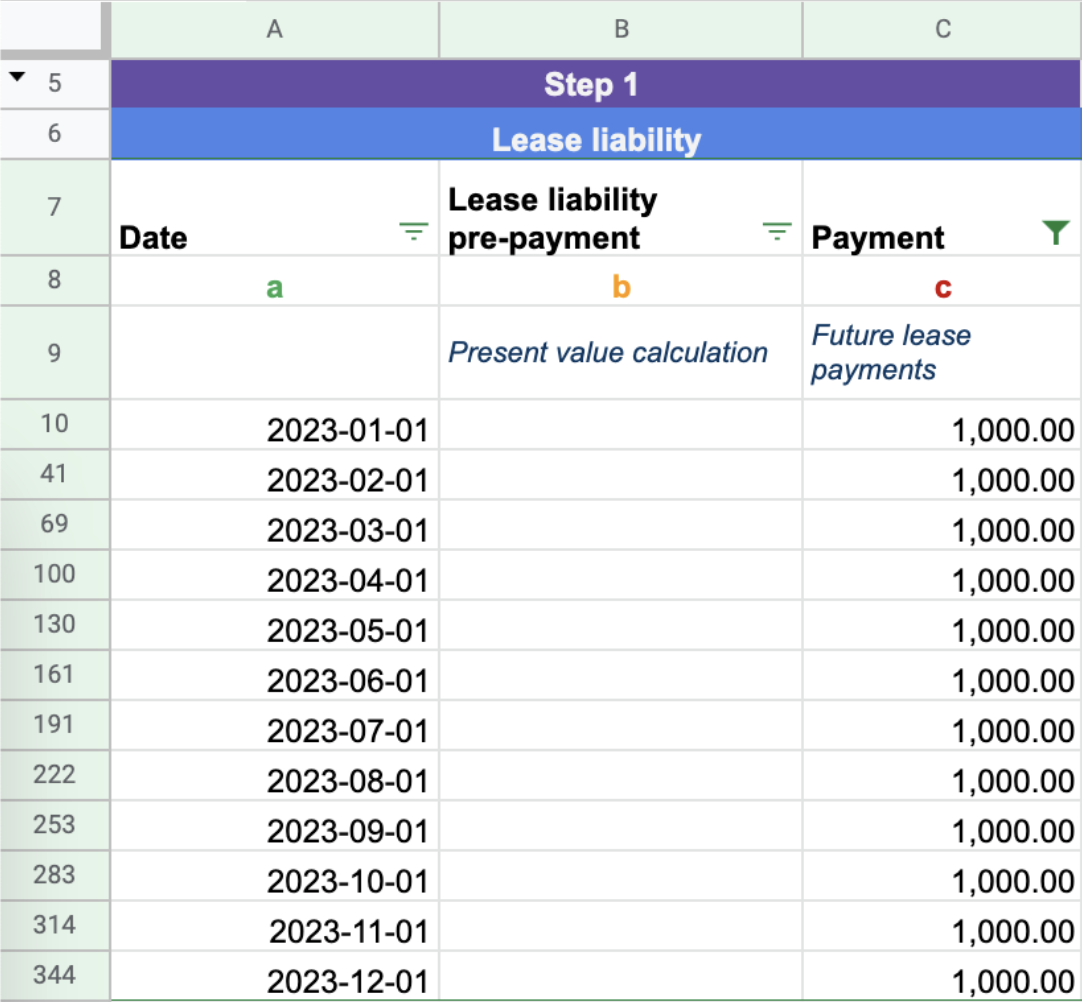These are the future payments related to the lease. Once these payments are present valued, it forms the lease liability. In this example, there are 12 payments of \$1,000 paid at the start of each month.

## Step 2 - Apply the discount rate and calculate the lease liabilityThis discount rate is one of the most subjective areas when accounting for a lease under GASB 87. On most occasions a discount rate will not be provided in the lease. In addition, if a discount rate is provided, that doesn’t necessarily mean it’s the appropriate rate to use.

GASB 87 offers two methods when calculating the discount rate. The rate implicit in the lease and if that amount is not readily determinable the incremental borrowing rate.

These following two articles, although geared towards ASC 842 use the exact same principles for GASB 87. As a lessee if you’re looking to determine the rate implicit in the lease, refer here on how to do that..

Alternatively, if you want to calculate the incremental borrowing rate of the lease, refer here.

In step one, we listed the future known lease payments and the date of each payment. As shown above the discount is the third input to present value the lease payments to calculate the lease liability.

In this example, we are using the XNPV function as it’s the most accurate present value calculation, compared to the NPV or PV functions in Excel. If you’re curious about the different present calculator methodologies we explore each one in greater detail here.

For Example 1, the inputs are:

• Rate: 7%
• Values: twelve \$1,000 payments at the start of each month
• Dates: 2023-01-01 to 2023-12-31

### Step 4 - Calculating the Unwinding of the Lease Liability to Zero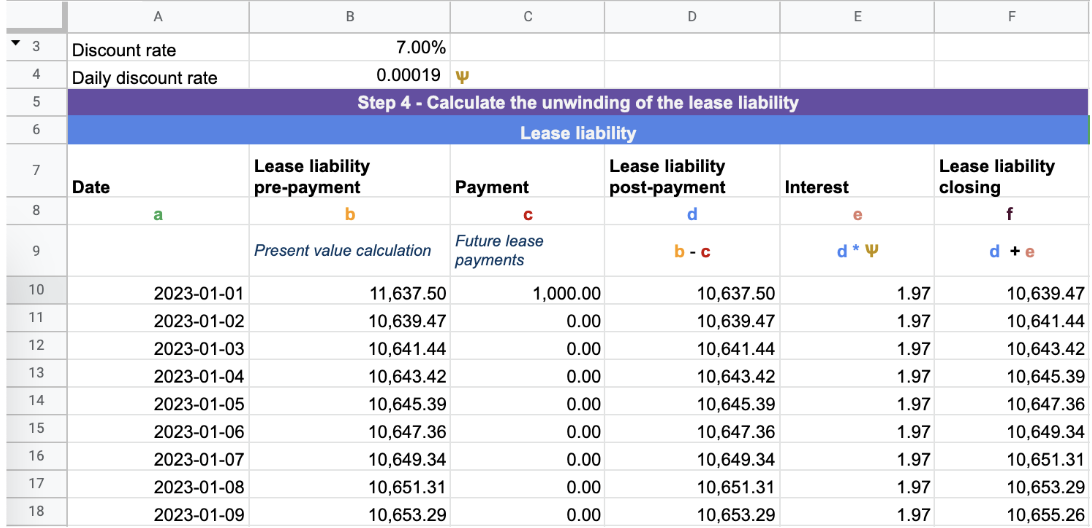Lease liability starts with an opening balance of \$11,637, which is expected to unwind completely over the life of the lease. As the lease liability accrues interest, the balance will increase, but lease payments will decrease the balance. It's important to note that the lease liability should always unwind to zero upon the expiry of the lease.

We have all the necessary inputs to calculate the unwind process from the present value at the start of the lease. If the lease liability does not unwind to zero, it's an indication of a miscalculation that needs to be addressed.

To break down the formulas used in each column, let's proceed.

Column A - Date - This encompasses every day within the lease agreement. For instance, in this particular scenario, the lease period starts from January 1st, 2023 and ends on December 31st, 2023.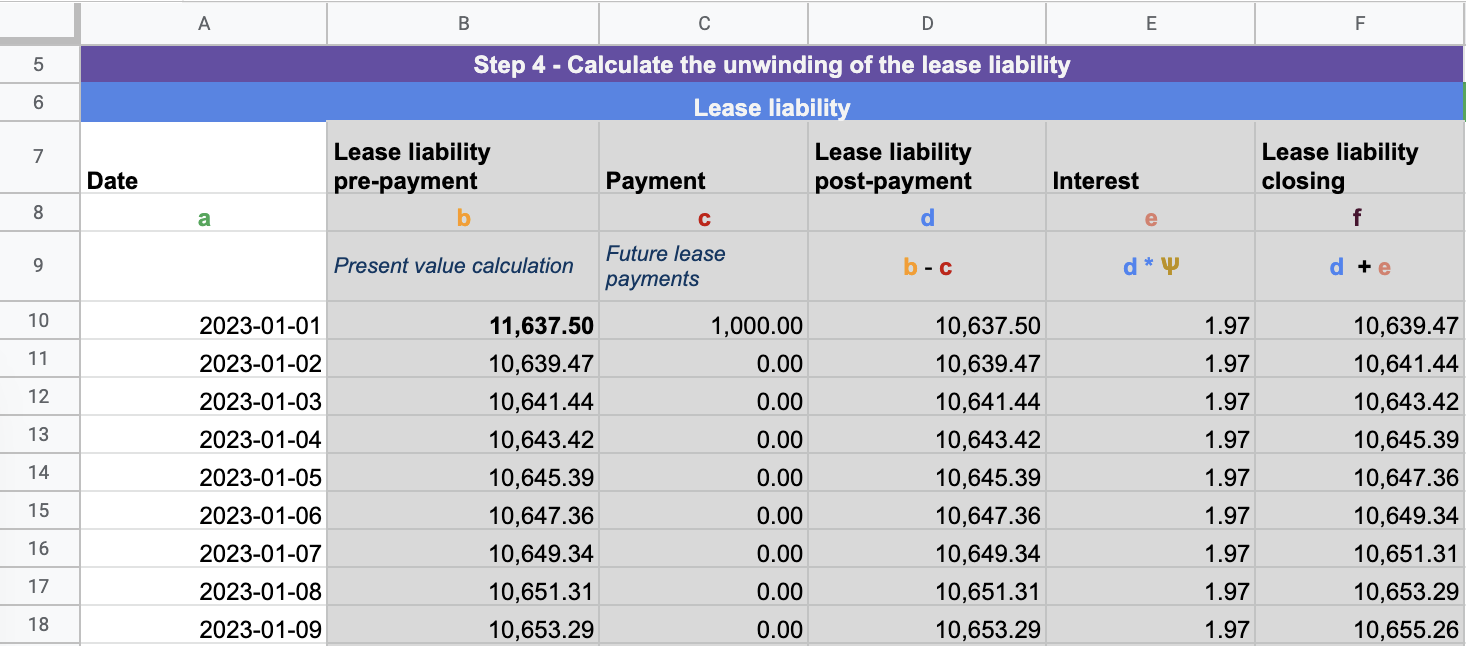Column B - Lease liability prepayment - In this column, you will enter the XNPV formula for each row. It's important to note that there are certain inputs in the calculation that are constant, such as the discount rate, the ending cash flow amount, and the end date.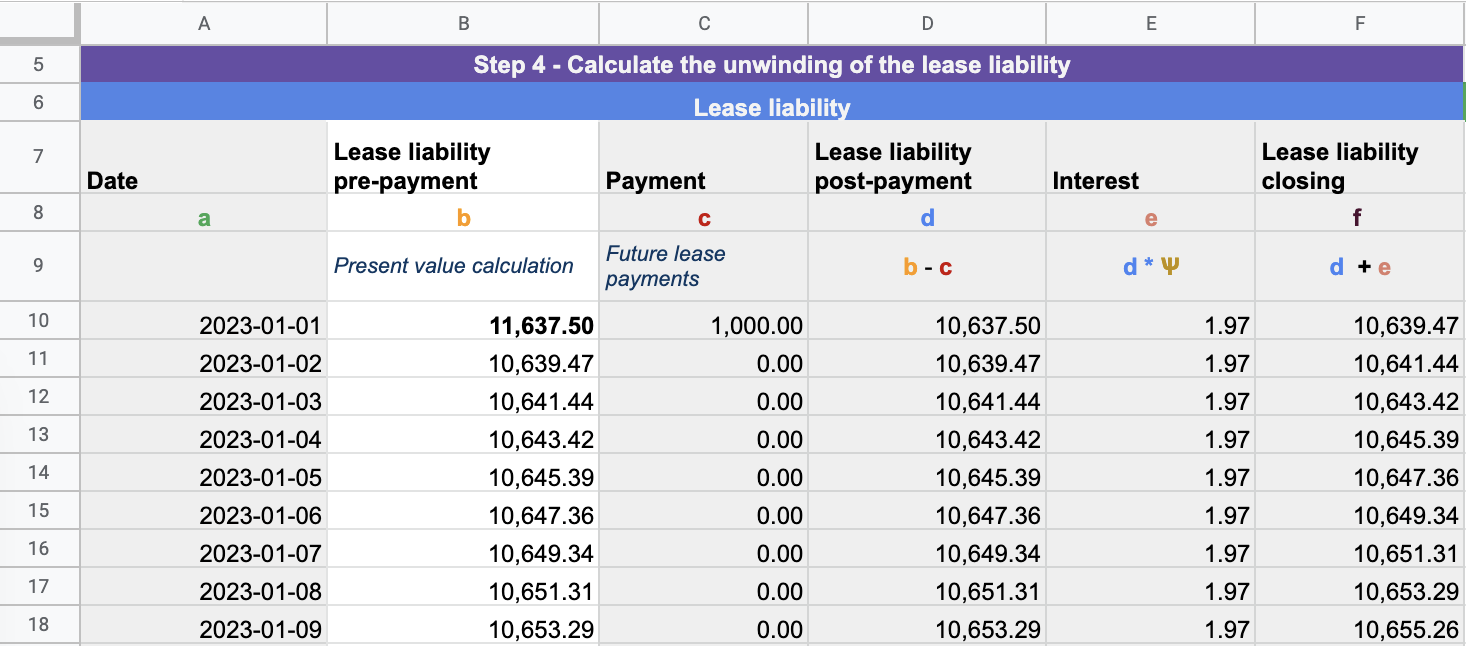Column C - Payment - The lease payments at each particular date: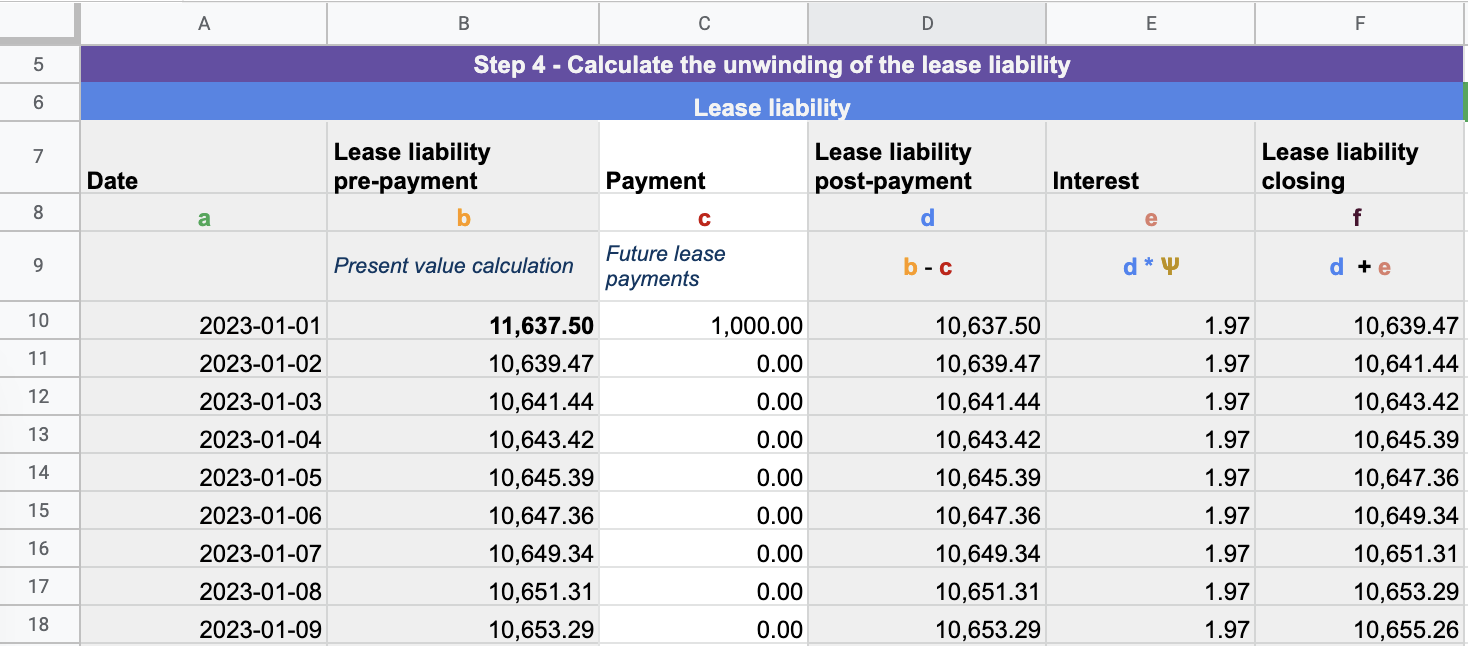Column D - Lease liability post-payment - This is the lease liability balance post payments. Each payment reduces the lease liability amount.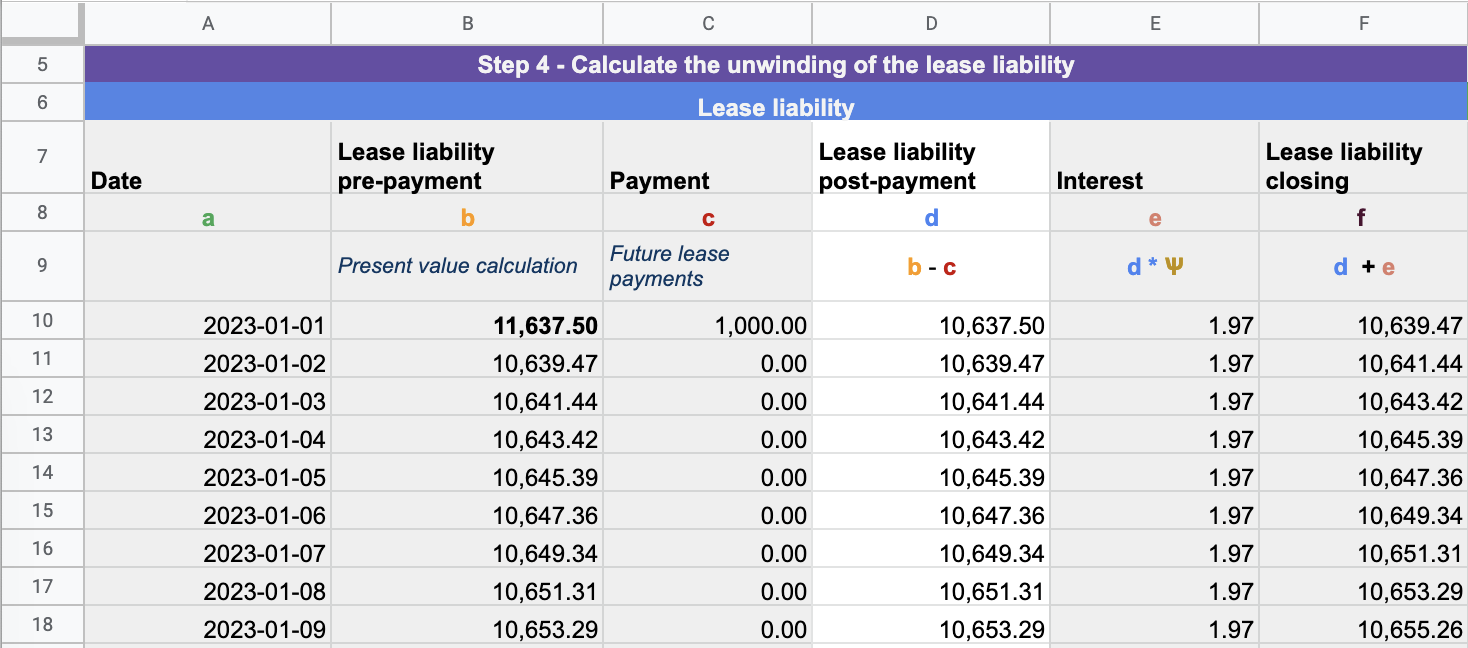Column E - Interest - This column represents the daily interest calculated on the lease liability using the daily discount rate.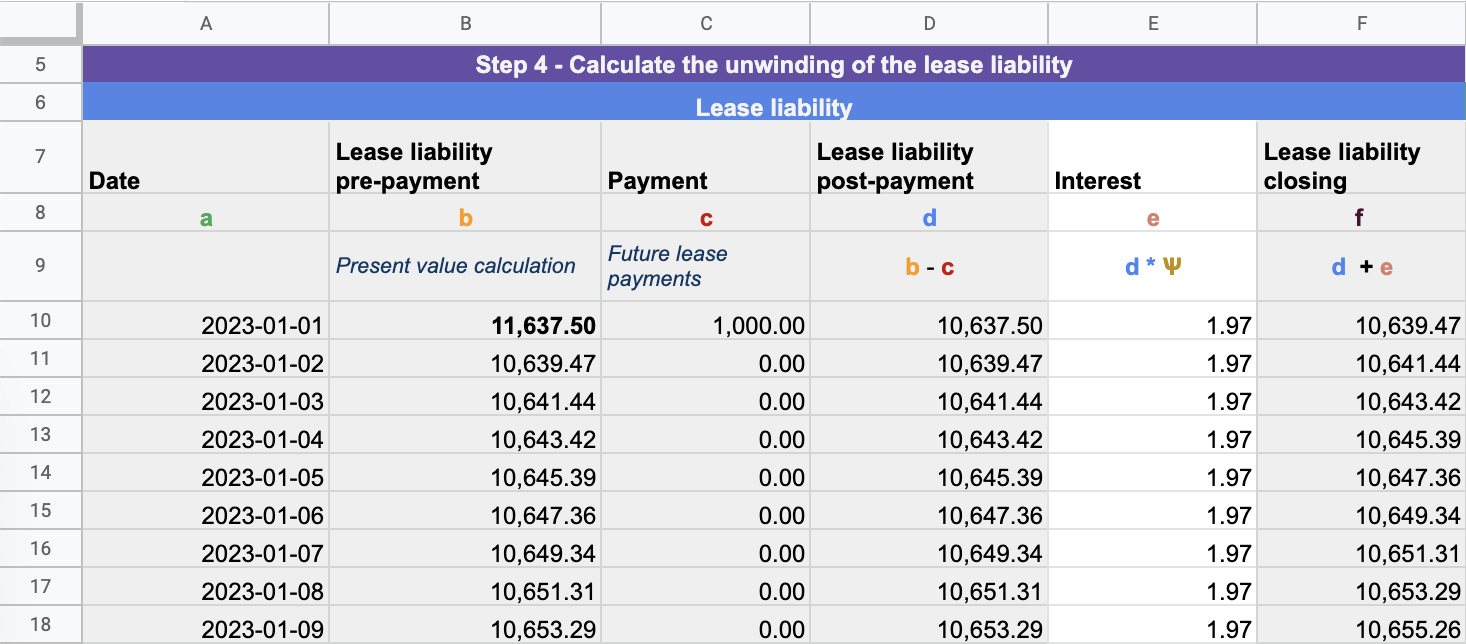To formula used to calculate the daily discount rate is the following:  (1+discount rate)^(1/365)-1

Column F - Lease liability closing - This column reflects the closing balance of the lease liability for the day, which incorporates the accrued interest. This closing balance serves as the opening balance for the subsequent day.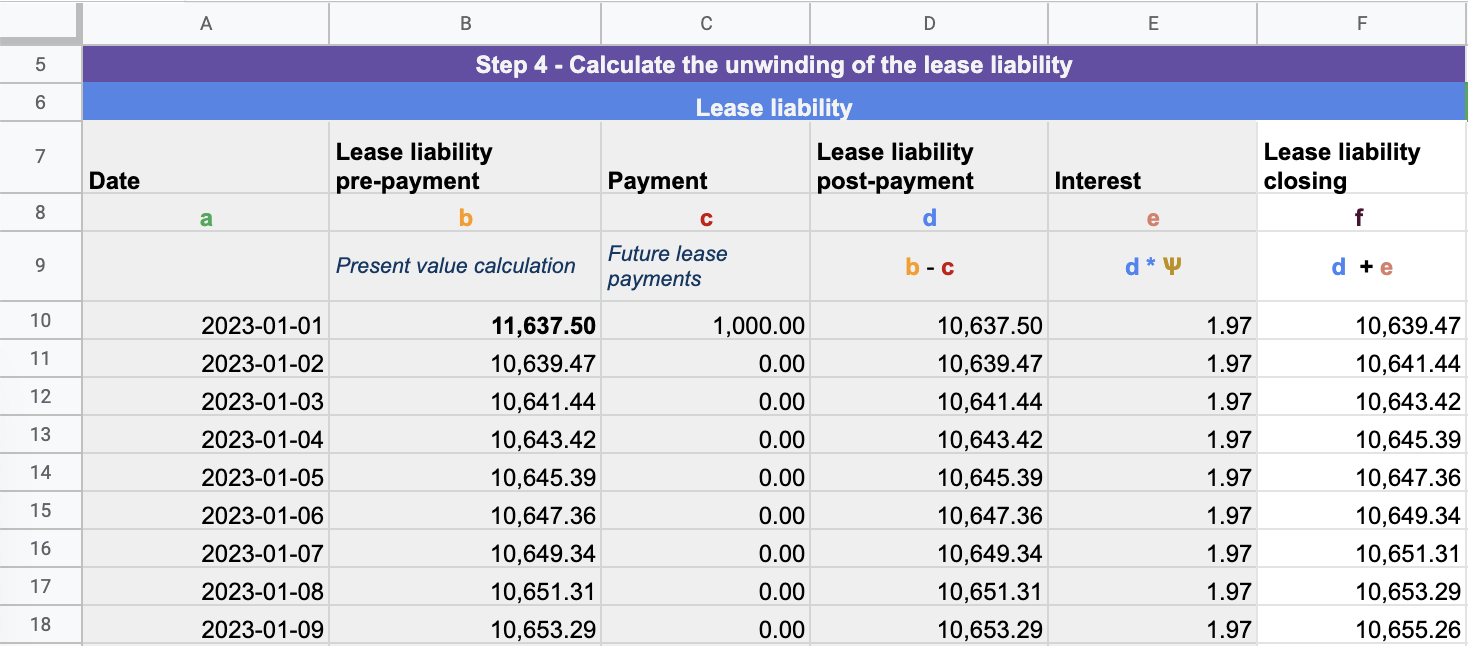The final test is to ensure the lease liability unwinds to zero: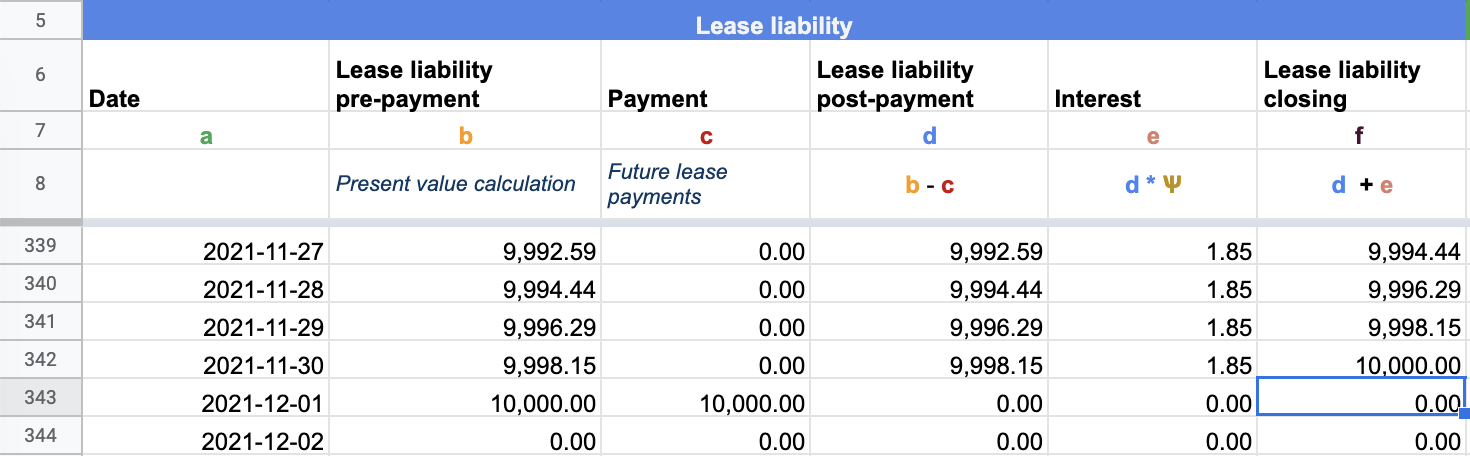## Step 5 - Calculating the Depreciation of the Lease Asset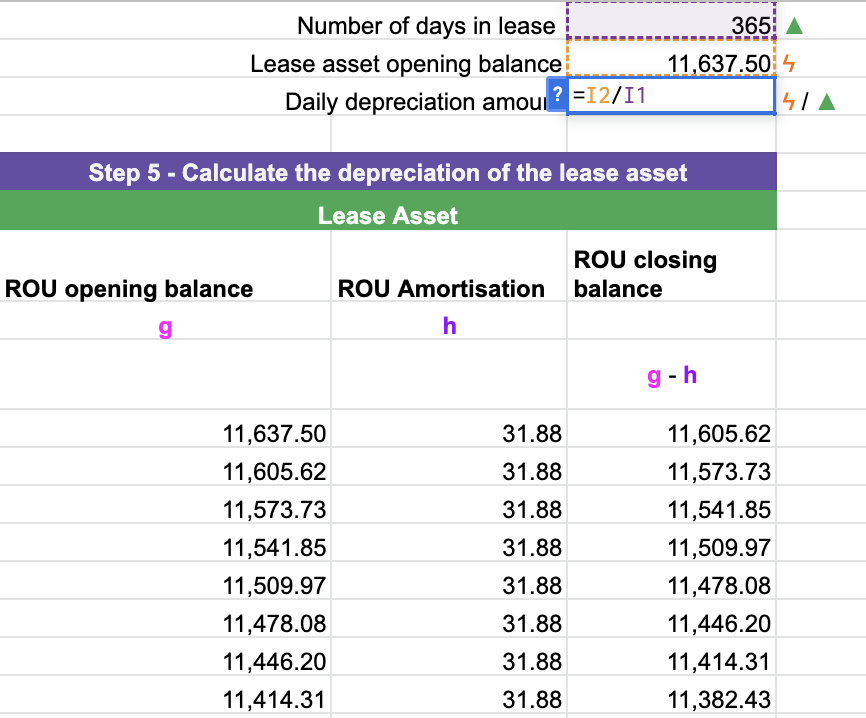After determining the lease liability, the next step is to calculate the depreciation of the lease asset. According to the standard, a lessee can use any systematic method to calculate depreciation. However, the simplest and most direct approach is the straight-line calculation method.

To calculate the straight-line depreciation, divide the opening balance of the lease asset by the total number of days in the lease. This will give you the daily depreciation rate. The following steps can be taken to perform this calculation:

• Determine the opening balance of the lease asset.
• Divide the opening balance by the total number of days the asset will be used. In most cases, this will be the end date of the lease.

From this calculation you have calculated the depreciation amount for the lease asset.

Update the calculation to deduct the depreciation amount from the lease asset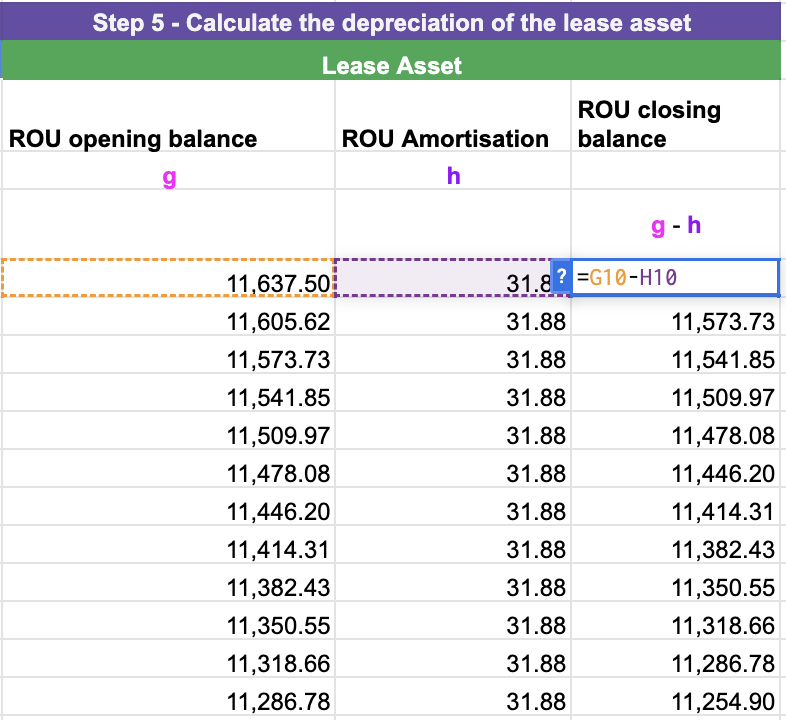Ensure the lease asset depreciates to zero: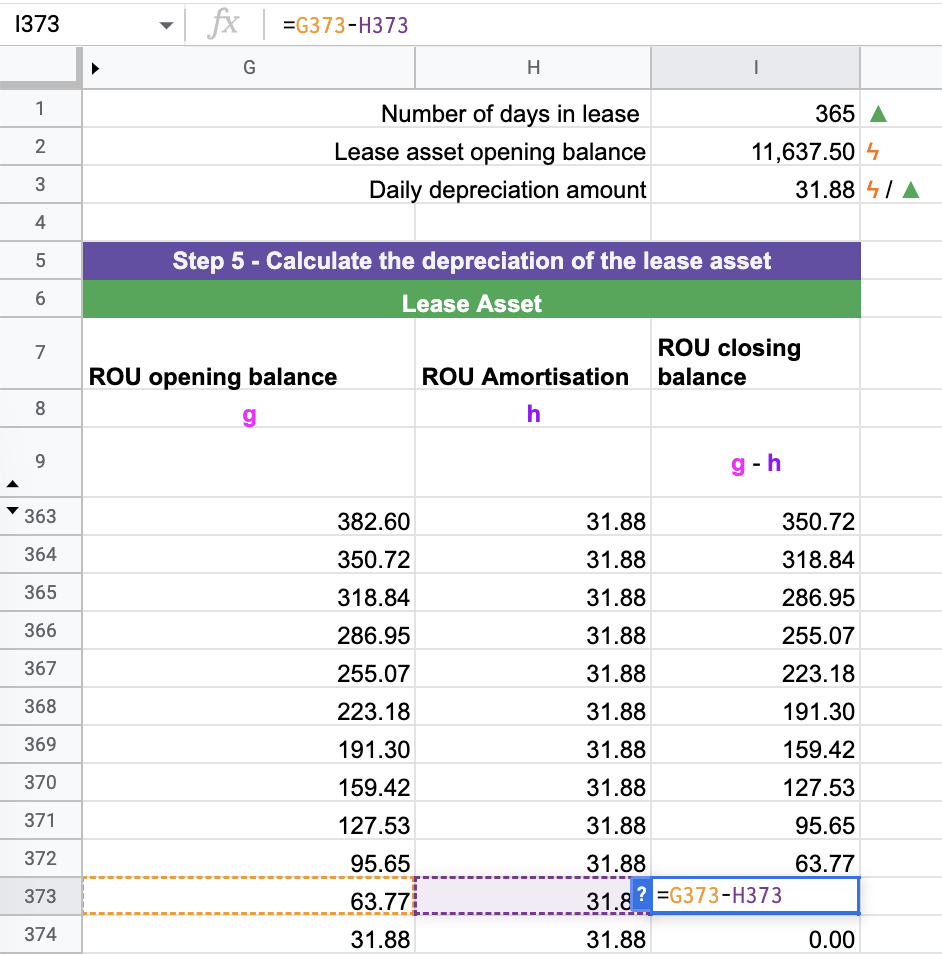Assuming that there have been no modifications to the lease, you have successfully computed a lease in accordance with GASB 87.

## Example 2 - Modification accounting

This example illustrates how to handle a lease modification in accounting. A modification could entail changing one or both of the following inputs:

• Lease payments
• Lease term

Whenever there is a modification, the lease liability must be remeasured based on the updated future lease payments. Generally, modifications involve either an increase or decrease in scope, which is also known as a partial termination. In the case of a decrease in scope modification, such as a reduction in the asset size or lease term, an additional gain/loss calculation is necessary.

In Example 2, we will walk through the steps required when a lessee decides to extend the lease term.

Using the details of Example 1:

• Start date: 2023-1-1
• Accounting End date: 2023-12-31
• Fixed Payment Amount: \$1,000
• Payment Frequency: Monthly
• Discount rate: 7.00%

Modification to the terms:

• On 2023-06-01, the lessee decided to exercise a renewal option until 2025-12-31
• The discount rate at modification is 5.00%

## Step 1 - Update the future lease payments from the date the modification is effective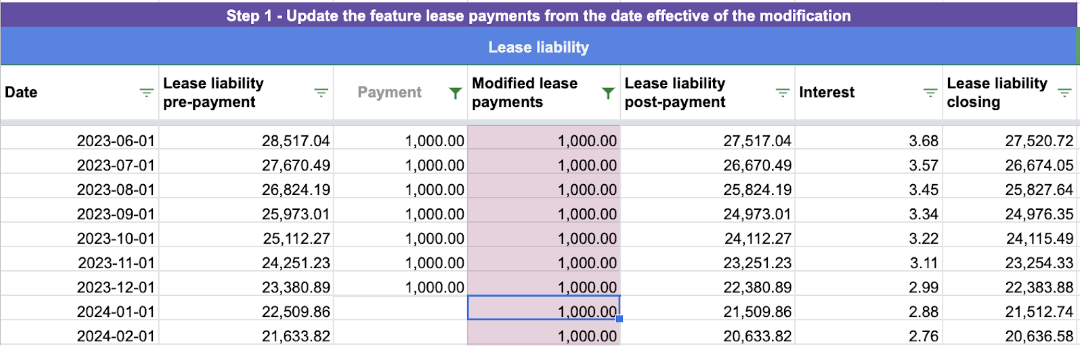When a modification occurs, an additional column is required to calculate the updated future lease payments. If you were to update the original column, it would then change the initial net present value calculations. This is something you do not want. For Example 2, there is an additional two years of lease payments for the year 2024 and 2025.

## Step 2 - Determine the appropriate discount rate and re-calculate the lease liability on the date effective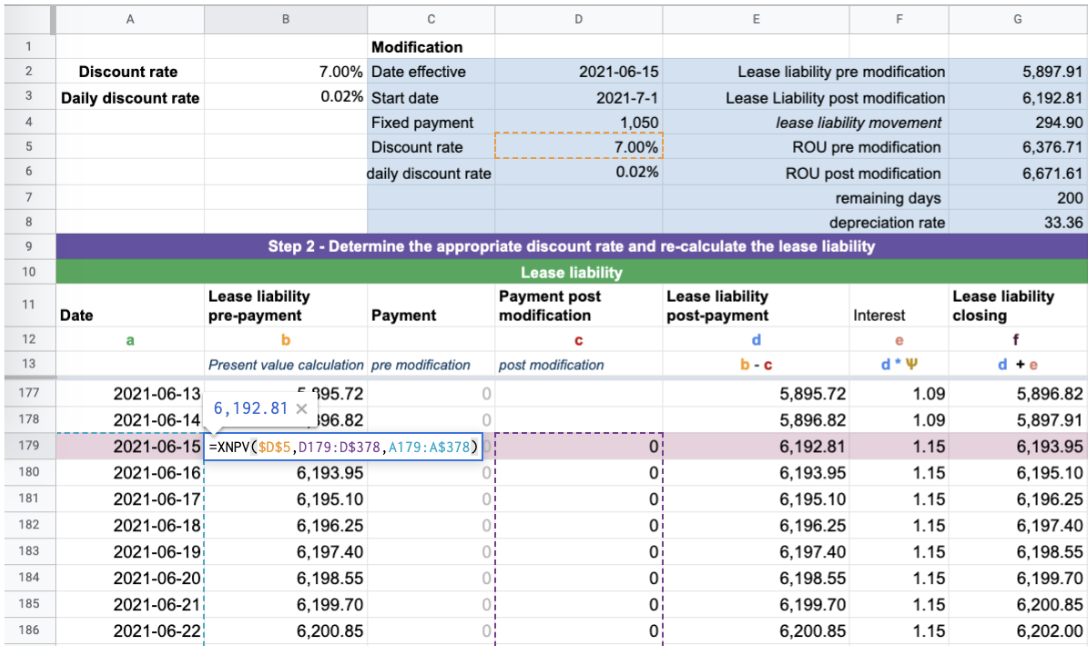This is where a solid understanding of modification accounting is required. There are two critical components to this calculation:

1) The remeasurement of the lease liability occurs on 2023-06-01. That’s when the lessee decided to exercise the renewal option until 2025-12-31. Because of this change, the lessee has a larger lease liability because of the increase in the future lease payments which occur over an additional two years.

2) In this example, the lessee is also required to recalculate the discount rate

In reference to Example 2, you can see that the XNPV present value calculation has been updated to reflect lengthening the lease.. In doing so, this changes the value of the lease liability.

a) Lease liability post payment will subtract payments from column D as opposed to column C: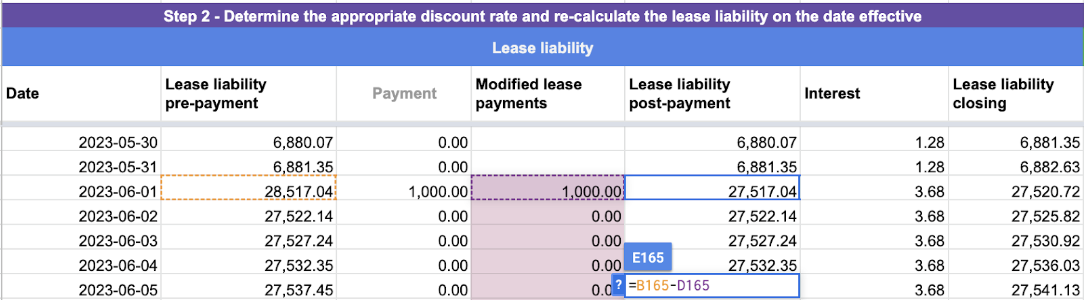b) Daily interest calculation will use the updated daily discount rate: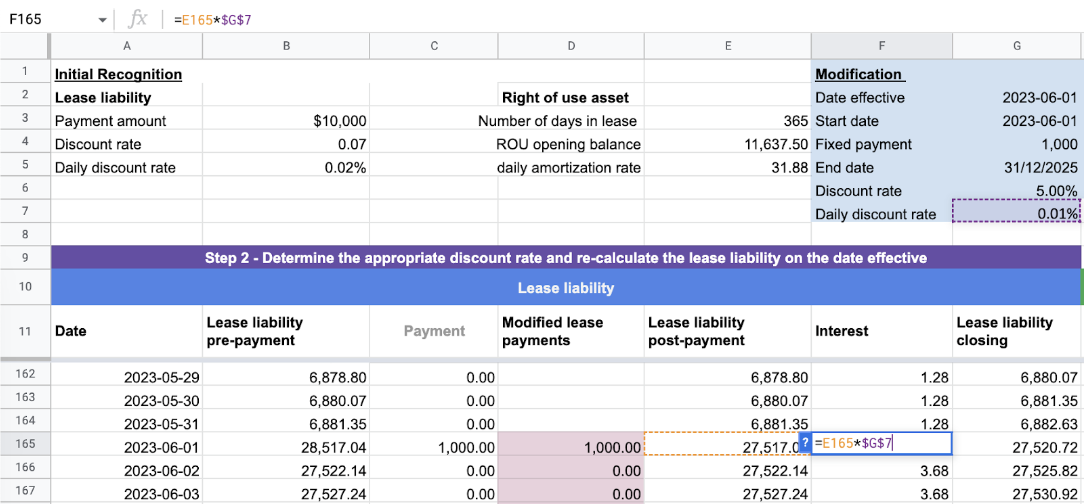Once you have made the following changes, ensure that the modified lease liability unwinds to \$0 based on the updated inputs.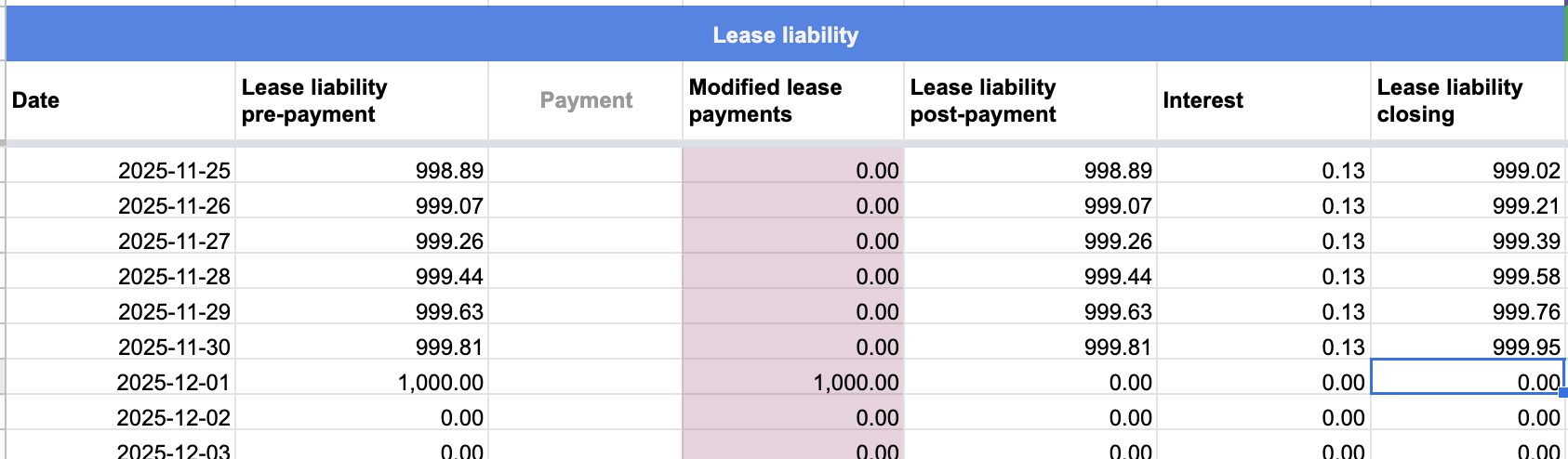In the event that the lease liability balance does not reach zero, it is likely due to one of the updates mentioned above not being applied. It is important to note that each row of the calculation must have these updates applied to it.

## Step 3 - Update the lease asset value based on the modification amount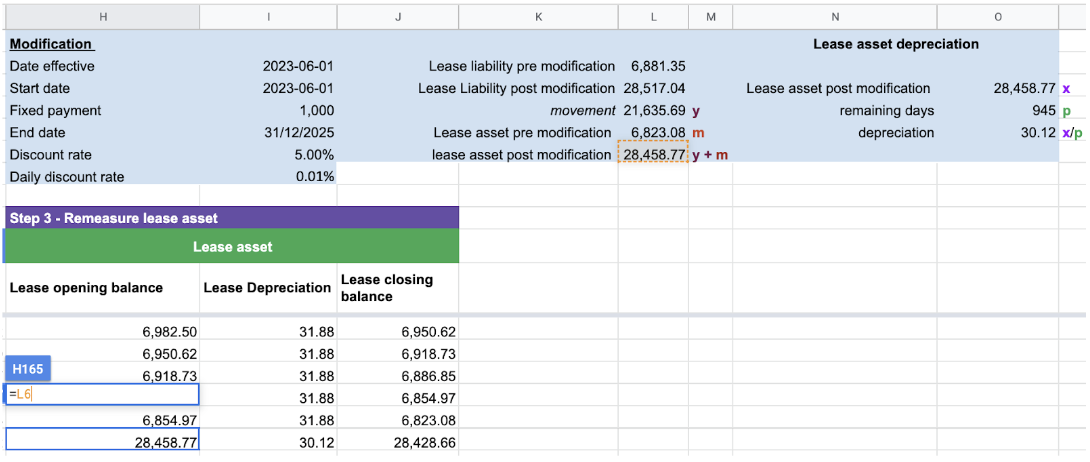The initial step involves calculating the movement of the lease liability pre and post-modification. For instance, in Example 2, the lease liability amount increased by \$21,635.69. Prior to modification, the lease liability stood at \$6,882.63, while post-modification, it increased to \$28,517.04.

To increase the lease liability, a credit entry is made, but the corresponding debit entry is made to the right of use asset as follows:

Debit - Lease Asset: \$21,635.69

Credit - Lease Liability: \$21,635.69

## Step 4 - Update the depreciation rateIf the value of the lease asset changes, the depreciation rate should be updated to ensure that the asset depreciates to zero by the end of its useful life. To determine the updated depreciation rate, divide the post modification value of the lease asset by the remaining days of its useful life. For Example 2, the calculation would be 28,458.77 divided by 945 the remaining days of the lease, resulting in a depreciation rate of \$30.12. It is important to verify that the updated depreciation rate ensures the asset depreciates to zero.

## Conclusion

After completing the last check, your work is finished! You may also find the following articles useful:

• A guide to GASB 87
• Article on how to calculate the discount rate
• A discussion on whether to comply with the lease accounting standard manually or by using lease accounting software

If you have made errors while performing the calculations in this article, there is a risk of manual error inherent in any manual process. One option to reduce this risk is to automate these calculations using lease accounting software, such as Cradle. By automating the process, you can minimize the chance of errors and ensure accuracy in your calculations.

## FooterHere at Cradle, our mission is simple; it's at the foundation of everything that we do. We want to make accountants' lives easier by leveraging technology to free up their time to focus on running the business.

140 Yonge St.
Suite 200
Toronto, ON M5C 1X6

US
Suite #73591
Torrance, CA 90503
USA

### Legal